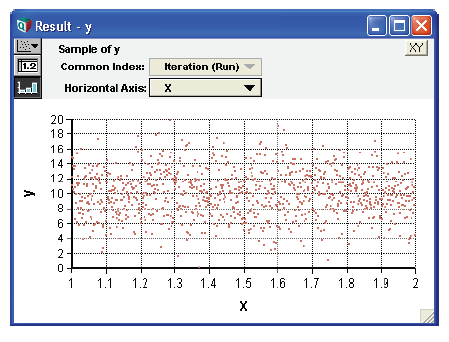# Scatter plots

A scatter plot graphs the samples of two probabilistic variables against each other, and provides insight into their probabilistic relationship.

To generate a scatter plot for two variables, x and y:

1. Open a Result window for y.
2. Click the XY button located in the top-right corner of the window to open the Object Finder dialog.
3. In the XY Comparison sources dialog, check Use Another variable.
4. Press the Add... button, and in the Object Finder dialog, select the x variable. Press OK twice.5. In the Uncertainty View popup menu (at the top-left of the Result window), select the Sample view.

If the variables are independent, the scatter plot points fall randomly on the graph. If the variables are totally dependent, the scatter plot points fall along a single line. The strength of the relationship is indicated by the degree to which the points are close to a line. If the line is straight, the relationship is linear; if the line is curved, the relationship is nonlinear.

You can superimpose several scatter plots of y in an array of uncertain quantities depending on x. The different quantities are represented by differently colored dots or symbols.

Example:

x: Uniform(1, 2)
y: Normal(10, 3)

The resulting scatter plot of two independent variables is shown below.